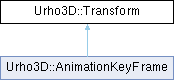Rebel Fork Framework
Urho3D::Transform Struct Reference

`#include <Urho3D/Math/Transform.h>`

Inheritance diagram for Urho3D::Transform:## Public Member Functions

Matrix3x4 ToMatrix3x4 () const
Construct Matrix3x4 from Transform.

Transform Lerp (const Transform &rhs, float t) const
Interpolate between two transforms.

Transform Inverse () const
Return inverse transform. It is not precise for non-uniform scale.

Transform operator* (const Transform &rhs) const
Return transform multiplied by another transform.

Vector3 operator* (const Vector3 &rhs) const
Return position multiplied by the transform.

Quaternion operator* (const Quaternion &rhs) const
Return rotation multiplied by the transform.

## Static Public Member Functions

static Transform FromMatrix3x4 (const Matrix3x4 &matrix)
Construct Transform from Matrix3x4. It is not precise for non-uniform scale.

## Public Attributes

Vector3 position_

Quaternion rotation_

Vector3 scale_ {Vector3::ONE}

## Static Public Attributes

static const Transform Identity {Vector3{}, Quaternion{}, Vector3{1.0f, 1.0f, 1.0f}}

## Detailed Description

3D transform decomposed into translation, rotation and scale components. TODO: Expand it into something more user-friendly.

The documentation for this struct was generated from the following files:
• Urho3D/Math/Transform.h
• Urho3D/Math/Transform.cpp